Automatically update charted data

## Description

Use data linking to synchronize charts and their workspace variables. Linked charts automatically update with changes to workspace variables, and workspace variables automatically update with chart modifications made via the brush tool.

Link charts and workspace variables using the `linkdata` function or by selecting the buttonfrom the figure toolbar.

## Creation

### Syntax

``linkdata on``
``linkdata off``
``linkdata``
``linkdata showdialog``
``linkdata(fig,___)``
``l = linkdata``
``l = linkdata(fig)``

### Description

example

````linkdata on` turns on data linking mode for the current axes.```
``linkdata off` turns off data linking mode.`
````linkdata` toggles the data linking mode between `'on'` and `'off'`.```

example

````linkdata showdialog` opens the Linked Plot Data Sources dialog box. Use this syntax to interactively synchronize a chart with workspace variables.```
````linkdata(fig,___)` specifies the data linking mode for the specified figure. Use single or double quotation marks around the options `'on'` and `'off'`. For example, `linkdata(fig,'on')`.```
````l = linkdata` creates a `LinkData` object. This syntax is useful for querying the data linking mode.```
````l = linkdata(fig)` creates a `LinkData` object for the specified figure.```

### Input Arguments

expand all

Target figure, specified as a `Figure` object. If you do not specify the figure, then data linking is enabled or disabled for the current figure.

## Properties

expand all

Data linking mode, specified as `'off'` or `'on'`.

## Examples

collapse all

Create a bar chart and enable data linking. Data linking synchronizes the bar chart with the `x` and `y` variables in the workspace.

```x = linspace(-5,5,11); y = abs(x); bar(x,y) linkdata on ```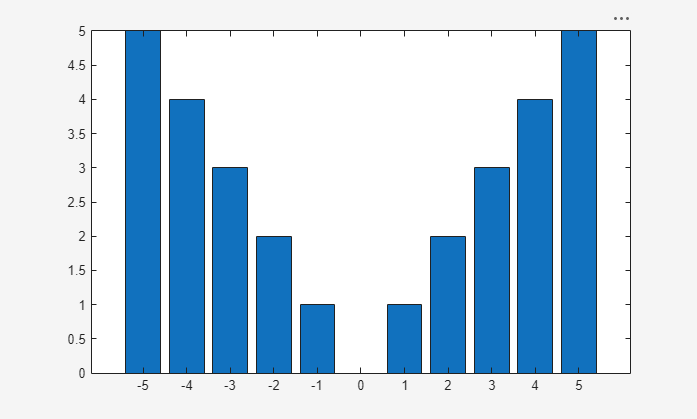Modify one of the values in `y`. Note that the bar chart immediately updates to reflect the change.

`y(6) = 4;`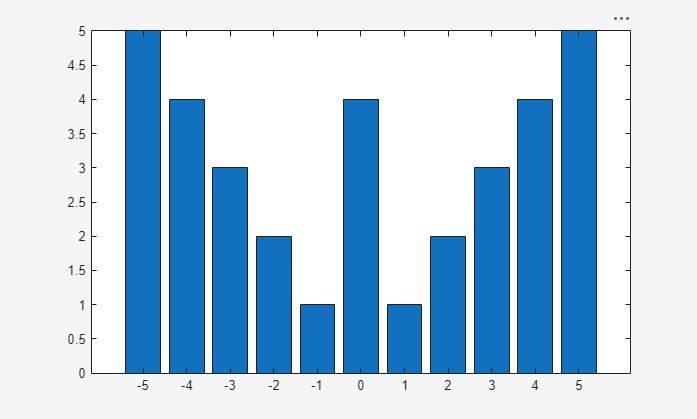Interactively delete workspace values by selecting them in a chart, using `linkdata` and the brush tool.

Create some data to plot. Note that `x` and `y` contain 21 values.

```x = linspace(-5,5,21); y = -abs(x); lengthX = length(x) lengthY = length(y)```
```lengthX = 21 lengthY = 21```

Plot the data, then enable both data linking and brushing.

```scatter(x,y,'filled') linkdata on brush on```

Highlight the data values you want to delete. To remove the brushed data from the chart, right-click on a brushed value and select Remove from the context menu.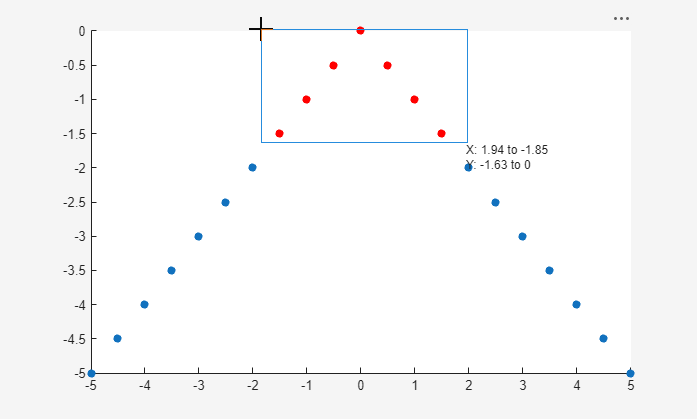The chart is linked to its data sources, so deleting values from the chart also deletes them from the data sources. Note that `x` and `y` now contain only 14 data values.

```lengthX = length(x) lengthY = length(y)```
```lengthX = 14 lengthY = 14```

Use data linking with complex numbers. When you plot complex data, the real and imaginary parts of the data do not correspond to different workspace variables. As a result, the `linkdata` function cannot identify data sources for the x- and y-axes. To link complex data, you must manually specify the real part of the complex data as the x-component and the imaginary part as the y-component.

First, create a matrix of complex data values and plot them. Then, open the Linked Plot Data Sources dialog box by calling ```linkdata showdialog```.

```z = eig(randn(20,20)); plot(z) linkdata showdialog ```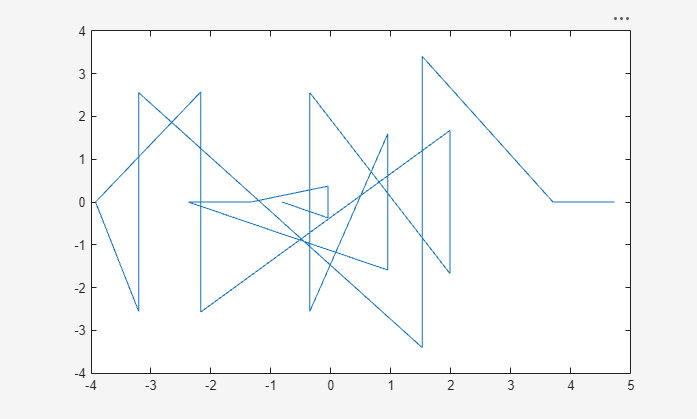Manually specify the real and imaginary parts of the data by typing `real(z)` and `imag(z)` in the text boxes under X and Y.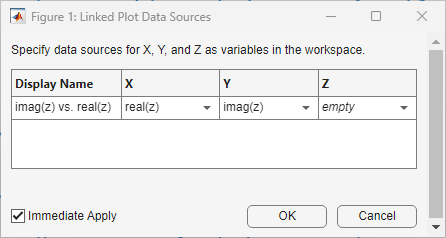Now the chart is linked to its data sources. If you change `z`, then the plot will update to reflect the new data.

To avoid using the dialog box, you can specify the data sources when first plotting the data values.

```z = eig(randn(20,20)); plot(z,'XDataSource','real(z)','YDataSource','imag(z)') linkdata on```

## Tips

• If `linkdata` cannot unambiguously identify data sources for a chart, then the chart will not synchronize with workspace variables. If you call `linkdata` and your chart does not update when you change a variable, then open the Linked Plot Data Sources dialog box by calling `linkdata showdialog` and manually link the chart to its data sources.

• The `linkdata` function updates linked data sources and charts around twice a second. To smoothly animate changes in data values, create loops that execute two times per second or less. For more information, see the `pause` function.

• Once a chart is synchronized with its workspace variables, programmatic changes to the data properties of the chart (for example, `XData`) do not update the workspace variables. Workspace variables only update when you modify plotted data interactively, using the brush tool.

## Algorithms

Data linking connects a chart and its workspace variables using the chart's data source properties (for example, `XDataSource`). When you turn on data linking for a figure, MATLAB® compares variables in the workspace to plotted data in the figure. When it finds a match, MATLAB assigns that variable to the appropriate data source property of the chart.

## Version History

Introduced in R2008a